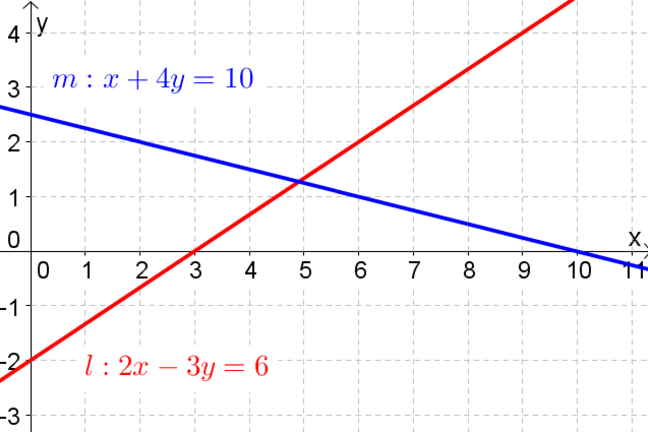## Want to keep learning?

This content is taken from the UNSW Sydney's online course, Maths for Humans: Linear and Quadratic Relations. Join the course to learn more.
2.9

## UNSW Sydney# Finding the meet of two lines

Finding the intersection, or meet, of two lines is simple geometrically–since the point where they cross is usually clear. However the same problem algebraically has an interesting history going back to ancient Chinese mathematicians.

In this step, you will see a clear cut algebraic strategy for solving a pair of general linear equations in $$\normalsize{x}$$ and $$\normalsize{y}$$.

## Solving a pair of equations

Suppose we want to solve the system

$\Large{4x-5y=14 \label{l1} \tag{1} }$ $\Large{3x+y=1. \label{l2} \tag{2}}$

Note that we have numbered the equations so we can refer to them. Since each equation represents a line, any point $$\normalsize{[x, y]}$$ satisfying both equations must lie on both lines. Thus solving this pair of equations is the same as geometrically finding the intersection of two lines. We look for an equation where we can conveniently solve for one of the variables. Conveniently here means with a minimum use of fractions! In $$\normalsize{(\ref{l2})}$$ we see that

$\Large{y=1-3x \label{l3} \tag{3}}$

Now we substitute that value of y into the other equation:

$\Large{4x-5(1-3x)=14}$

and simplify

$\Large{4x-5+15x=14}$ $\Large{19x=19}$ $\Large{x=1}.$

Having the value for $$\normalsize{x}$$, we go back to $$\normalsize{(\ref{l3})}$$ to find that

$\Large{y=1-3\times 1=-2}.$

So our solution is $$\normalsize{x=1}$$ and $$\normalsize{y=-2}$$, or geometrically the point $$\normalsize{[1,-2]}$$. As pictured!This is an important skill, so make sure you know how to do it by working through the following, if this is not already familiar to you.

Q1 (E): Solve $$\normalsize{5x-2y=7}$$ and $$\normalsize{x+7y=31}$$.

Q2 (E): Solve $$\normalsize{4x+y=39}$$ and $$\normalsize{8x+7y=53}$$.

Here are some questions that amount to the same thing, but are recast in different ways.

Q3 (E): Where do the lines $$\normalsize{y=4x-6}$$ and $$\normalsize{y=-x+9}$$ meet?

Q4 (M): I am thinking of two numbers, which add up to $$\normalsize{99}$$ and differ by $$\normalsize{31}$$. What are they?

A1. The solution is $$\normalsize{x=3}$$ and $$\normalsize{y=4}$$.

A2. The solution is $$\normalsize{x=11}$$ and $$\normalsize{y=-5}$$.

A3. The lines meet at the point $$\normalsize{[3,6]}$$.

A4. This question should first be rephrased as two linear equations. Let the two numbers be $$x$$ and $$y$$. Then $$x+y=99$$ and $$x-y=31$$. These can be solved together to deduce that $$x = \normalsize{65}$$ and $$y = \normalsize{34}$$.